# Quantum Algorithms

## The Quantum Fourier Transform

The QFT is used in many important quantum algorithms and it is important to understand it. It is analogous to the classical discrete Fourier transform but should be understandable to anyone that has understood the basics of quantum computation. It can be carried out in O(n2) time and is exponentially faster than the classical equivalent.

## Beware

Many resources have their least significant qubits at the bottom whereas in this site we position ours at the top. This is so the circuits we produce work with Quirk. Just bear in mind that other resources might organise their qubits in the reverse order (or ‘upside down’).

## Intuition

You will remember that classically we count through states using binary: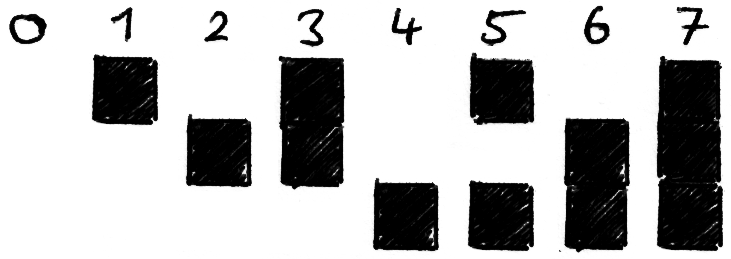When counting in binary, the uppermost bit toggles on/off with every increment, the next bit toggles on/off with every 2 increments and the third bit toggles on/off with every 4 increments. The rule is that every nth qubit toggles on/off after every 2n increments.

Being classical states, each state has no phase and the information (the number we are storing) is stored in the amplitudes of |0⟩ and |1⟩ for each qubit. Let us now introduce the Fourier basis. This is just another method of representing numbers, but instead using qubit phase: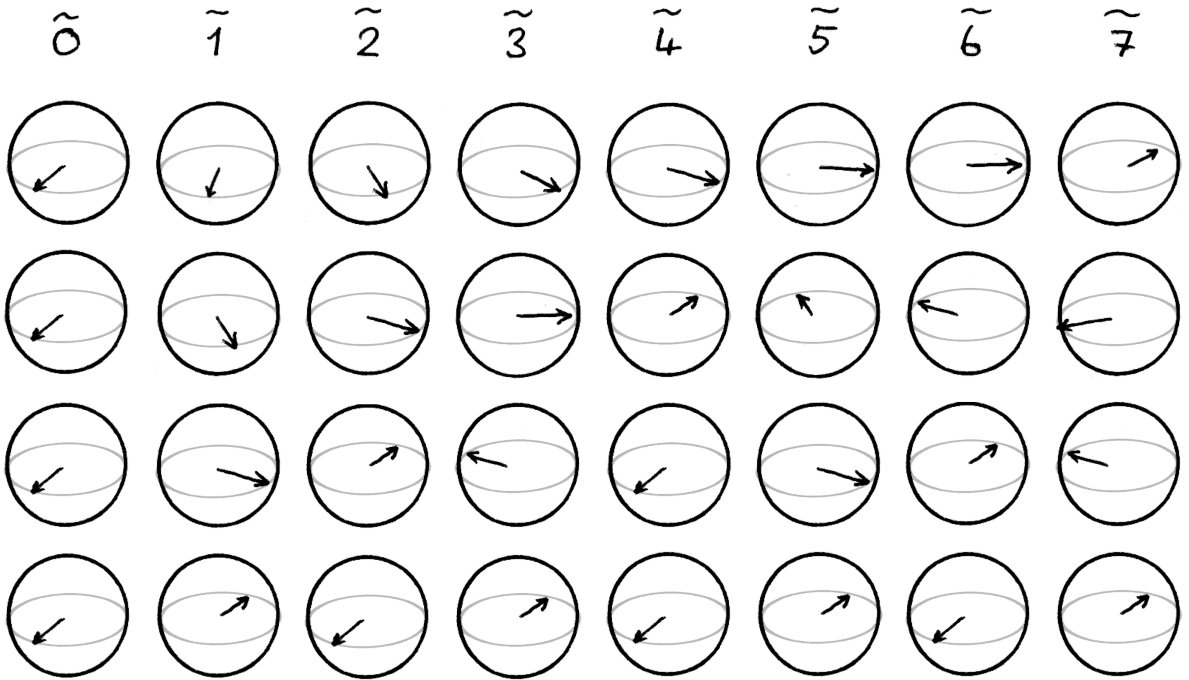(We differentiate states in the Fourier basis from the classical basis through the tilde (~) on top of the numbers). We can see that in the state ᷉0, all qubits are in the |+⟩ state. For each increment, we rotate the lowest qubit by π around the Z-axis of the Bloch sphere, the next qubit is rotated by π/2 for each increment, and the third by π/4. We see a related but different counting rule: the nth qubit is rotated by π/2n-1 for each increment. This may be easier to see as an animation: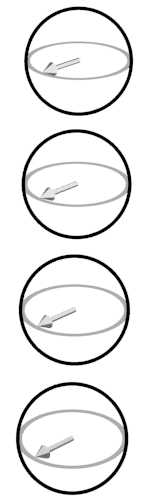The Quantum Fourier Transform (QFT) is simply the operation that transforms the classical number state to it’s corresponding Fourier state.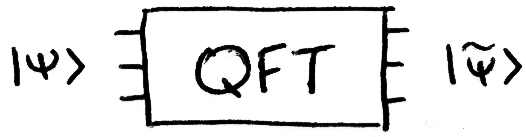### In Mathematical Notation

The QFT is often described by the formula: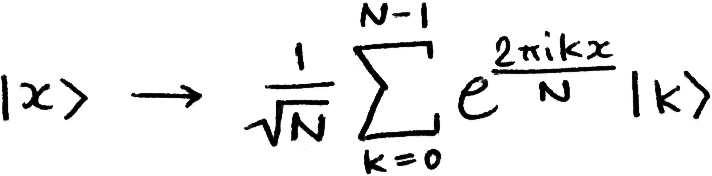There is another common way of writing in terms of the individual qubits. To do so we should refresh ourselves on how we convert binary fractions into decimal. Remember that the value of each digit halves as we move right, this carries on past the point in the same way as with decimal numbers: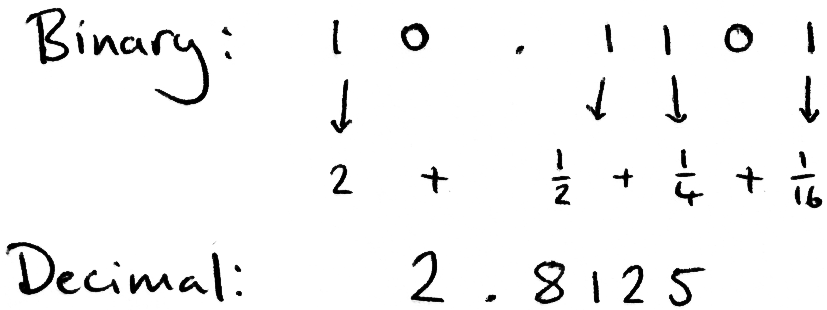We can then use this notation to write the QFT like this: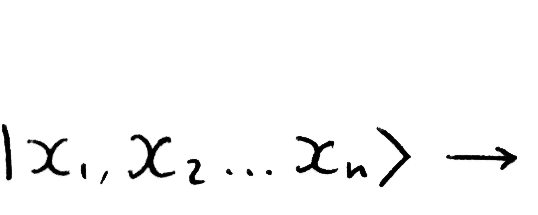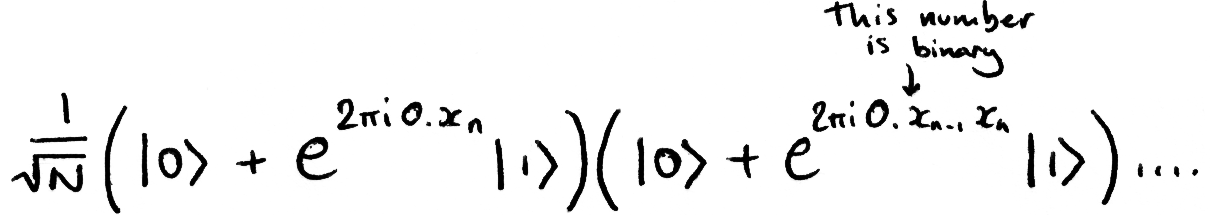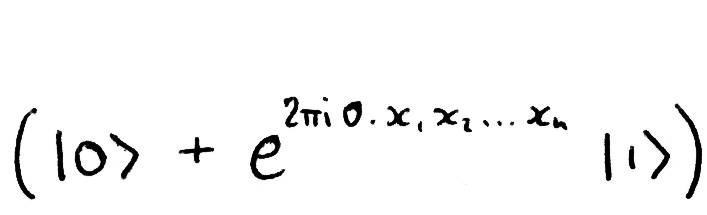To illustrate, let’s look at an example with three qubits: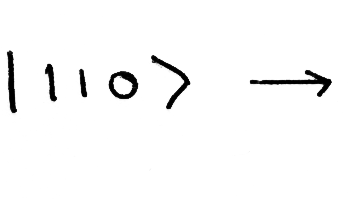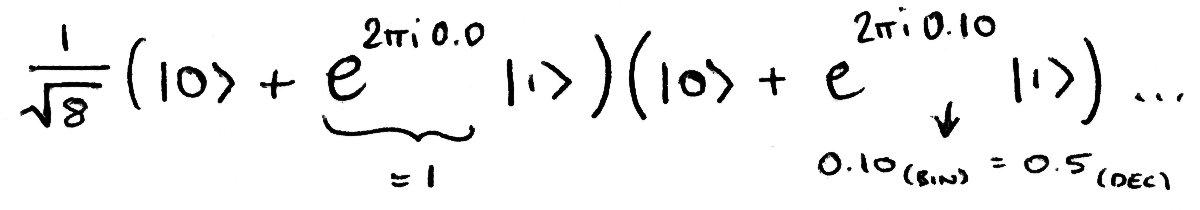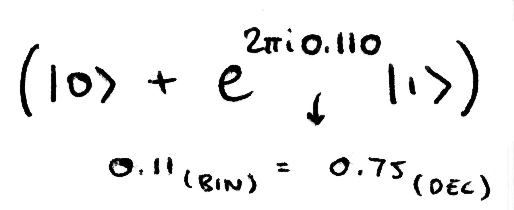110(binary) = 6(decimal). We can see that the QFT does indeed behave as described in the intuition section. In the Fourier state 6 we see that the bottommost qubit has a phase of 1 (no phase), the second has a phase of e (0.5 of a full rotation) and the top qubit has a phase of ei3π/2 (0.75 of a full rotation):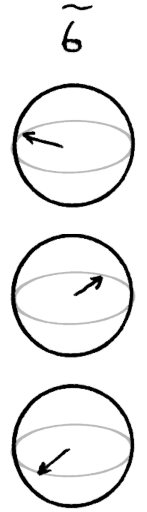## Building a Quantum Circuit

Next, lets try and create a quantum circuit that carries this operation out. We’ll start with three qubits, then generalise to any number of qubits.

Firstly, its easier to implement the QFT upside down, the reasons for this will become apparent as we create the circuit but until then we will put some swaps at the end of the transform to arrange the qubits in the correct order.

We need the output state of the uppermost qubit to switch between |+⟩ and |-⟩ as it’s input switches between |0⟩ and |1⟩. We can easily implement this with a H-gate, this is the one-qubit QFT: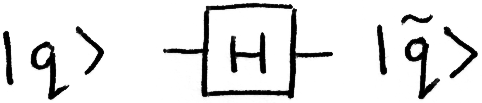Next, we need the second qubit to start in the state |+⟩ and rotate 90 degrees for each increment of our classical input. We accomplish this using a H-gate and a controlled-Rϕ gate, rotating by π/2 (also known as an S-gate). Note that we need to do this before applying the H-gate to the top qubit: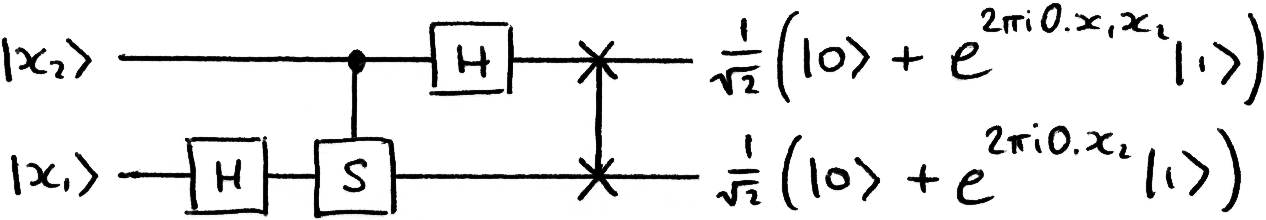We can verify that this works correctly for all 4 input states. Finally, we use another H-gate and two controlled-Rϕ gates (S & T) to correctly transform the final qubit: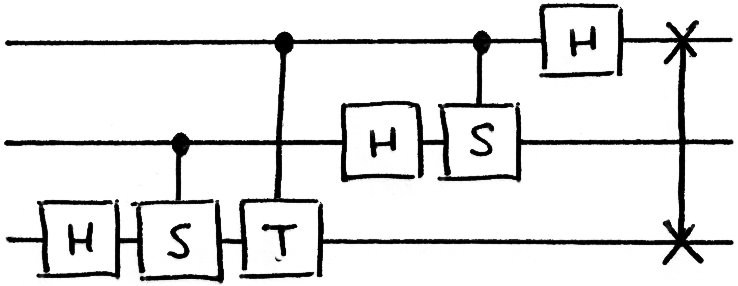Hopefully you have an idea as to how this scales to a larger number of qubits. Quirk has a nice demonstration of the QFT on 8 qubits. The general circuit for the QFT on n qubits looks like this: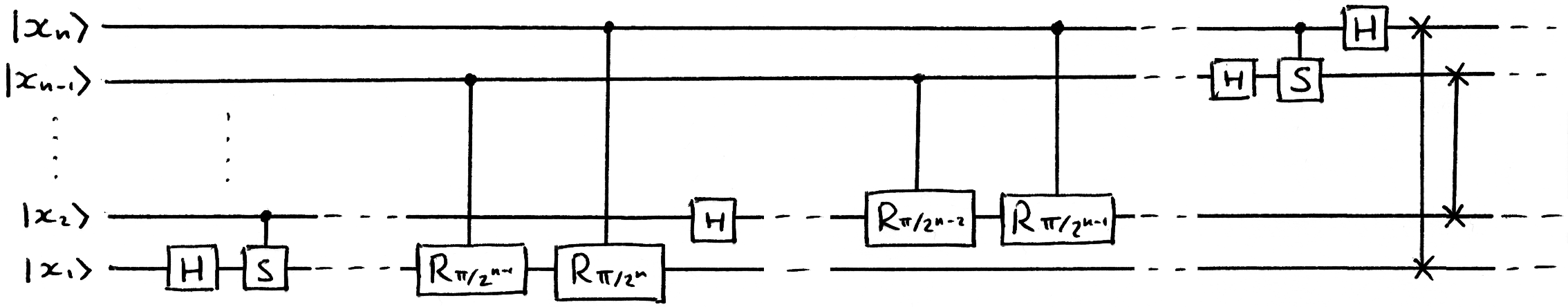Remember that an S-gate is an Rπ/2-gate and that we need to add the swaps at the end of the circuit.

### Approximate QFT

You might notice that for large circuits with many qubits, we are spending a lot of time doing tiny rotations. These tiny rotations have a tiny impact on the final state of the qubits and it turns out that we can ignore rotations below a certain threshold and still get decent results. Cutting out these gates naturally improves performance and gives us a simple approximate QFT. Approximate QFTs can be created with O(nlog(n)) gates, we will not cover this here but it is worth remembering.